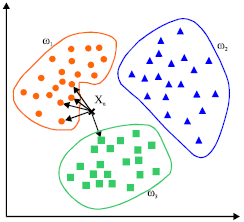# K-Nearest Neighbors (KNN)

Jump to: navigation, search

Can be used for regression or classification by choosing 'K'; where 'K' = # of closest neighboring data points that the model uses in prediction.

This is a simple algorithm which predicts unknown data point with its k nearest neighbors. The value of k is a critical factor here regarding the accuracy of prediction. It determines the nearest by calculating the distance using basic distance functions like Euclidean. However, this algorithm needs high computation power and we need to normalize data initially to bring every data point to same range. 10 Machine Learning Algorithms You need to Know | Sidath Asir @ Medium